# Quiz 11: Expenditure Multipliers: the Keynesian Model

Autonomous expenditure refers to summation of investment and government expenditure. In addition, consumption expenditure might have an autonomous portion in it. In other words, we can say that autonomous expenditure is that expenditure which a country incurs when GDP is zero. It means autonomous expenditure is that part of the aggregate expenditure, which does not depend on GDP. The components of aggregate planned expenditure of Turtle Island are given below: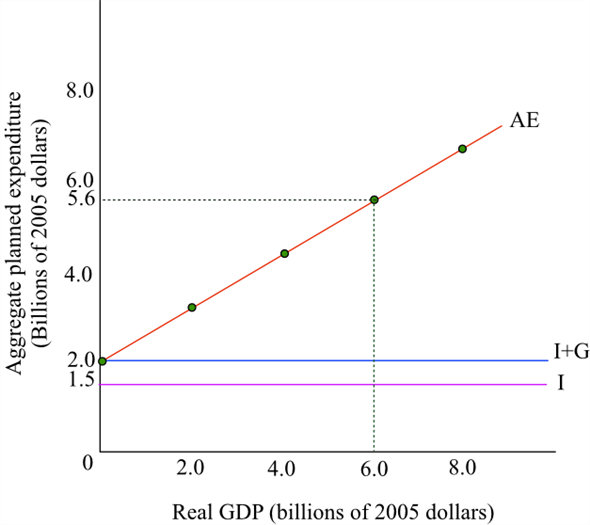Here autonomous expenditure is \$ 2 billion as it is the summation of investment and government expenditure. This is because, the autonomous consumption expenditure is zero here, and investment and government expenditure add up to \$ 2 billion. We know that marginal propensity to consume can be obtained by dividing the change in consumption expenditure by change in the disposable income.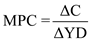Where: • MPC=Marginal propensity to consume •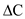=Change in consumption expenditure •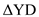Change in disposable income Now, substitute the values in the formula:Here marginal propensity to consume is 0.60.
The components of aggregate planned expenditure of Turtle Island are given below: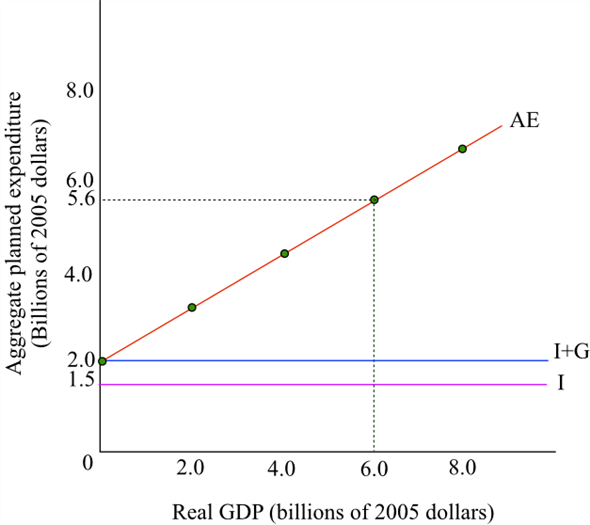a) Aggregate planned expenditure refers to the summation of expenditure on consumption, investment, and government expenses. From AE curve in the figure, we can get the aggregate planned expenditure corresponding to real GDP. Aggregate planned expenditure in case of GDP \$ 6 billion is \$ 5.6 billion. b) When the GDP is \$ 4 billion then AE expenditure is more than that. It means inventories are falling which implies firms will increase its production. c) In case real GDP is \$ 6 billion then aggregate planned expenditure is \$ 5.6 billion. It means that, aggregate planned expenditure is lesser than the GDP and it implies that inventories are rising. Moreover, firms will in turn, decrease its production.